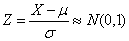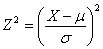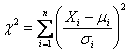# Exact Sampling distributions Assignment Help

## 11.1 Chi-Square Variate:The square of a standard normal variate is known as chi-square variate with 1 degree of freedom

Thus if X≈ N(μ, σ2), thenAndis a chi-square variate with 1 degree of freedom.

In general, if Xi, (i = 1, 2, ……, n) are n independent normal variates with mean μi and variance σi2, (i = 1, 2, ……, n), thenis a chi-square variate with n degrees of freedom.

## Exact Sampling distributions Assignment Help Through Online Tutoring and Guided Sessions from AssignmentHelp.Net